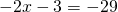Chapter 2: Linear Equations

# 2.2 Solving Linear Equations

When working with questions that require two or more steps to solve, do the reverse of the order of operations to solve for the variable.

Example 2.2.1

Solve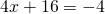for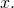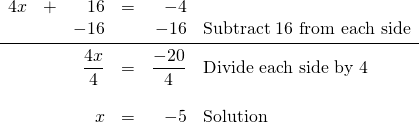In solving the above equation, notice that the general pattern followed was to do the opposite of the equation.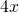was added to 16, so 16 was then subtracted from both sides. The variable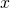was multiplied by 4, so both sides were divided by 4.

Example 2.2.2

1.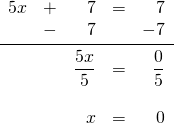2.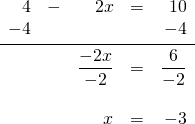3.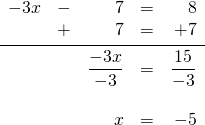# Questions

For questions 1 to 20, solve each linear equation.

1.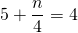2.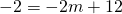3.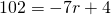4.5.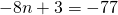6.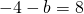7.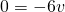8.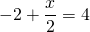9.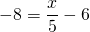10.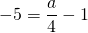11.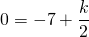12.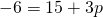13.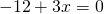14.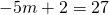15.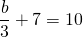16.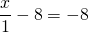17.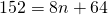18.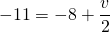19.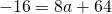20.